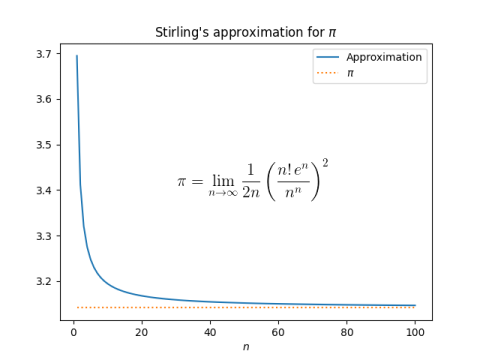# Calculating π with factorials

In honor of Pi Day, I present the following equation for calculating π using factorials.It’s not a very useful formula for π, but an amusing one. It takes a practical formula for approximating factorials, Stirling’s formula, and turns it around to make an impractical formula for approximating π.

It does converge to π albeit slowly. If you stick in n = 100, for example, you get 3.1468….You can find a more practical formula for π, based on work of Ramanujan, that was used for setting several records for computing decimals of π here.

You could code up the formula above in basic Python, but it will overflow quickly. Better to compute its logarithm, then take the exponential.

    from scipy.special import gammaln
from numpy import log, exp

def stirling_pi(n):
return exp(2*(gammaln(n+1) + n - n*log(n)) - log(2) - log(n))